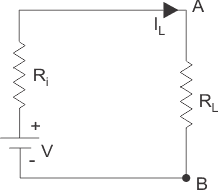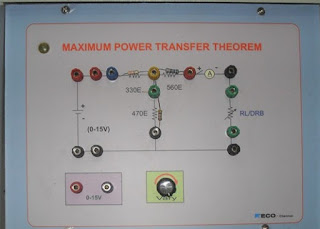Maximum Power Transfer Theorem Suppose we have a voltage source or battery that's internal resistance is R i and a load res...

## Maximum Power Transfer Theorem

Suppose we have a voltage source or battery that's internal resistance is Ri and a load resistance RL is connected across this battery. Maximum power transfer theorem determines the value of resistance RL for which, the maximum power will be transferred from source to it. Actually the maximum power, drawn from the source, depends upon the value of the load resistance. There may be some confusion let us clear it.Power delivered to the load resistance,To find the maximum power, differentiate the above expression with respect to resistance RL and equate it to zero. Thus,Thus in this case, the maximum power will be transferred to the load when load resistance is just equal to internal resistance of the battery.

Maximum power transfer theorem can be applicable in complex network as follows-
A resistive load in a resistive network will abstract maximum power when the load resistance is equal to the resistance viewed by the load as it looks back to the network. Actually this is nothing but the resistance presented to the output terminals of the network. This is actually Thevenin equivalent resistance as we explained in Thevenin's theorem if we consider the whole network as a voltage source. Similarly, if we consider the network as current source, this resistance will be Norton equivalent resistance as we explained in Norton theorem.Name

BASIC ELECTRICAL,12,BATTERIES,4,CIRCUIT THEORIES,9,CONTROL SYSTEMS,3,DC MOTOR,1,DIGITAL ELECTRONICS,1,DISTRIBUTED GENERATION,2,DISTRIBUTION,6,ELECTRICAL DRIVES,1,ELECTRICAL LAWS,8,ELECTRONICS DEVICES,2,General,7,GENERATION,3,GENERATOR,1,HIGH VOLTAGE,4,ILLUMINATION,1,INDUCTION MOTOR,7,MATERIALS,1,MEASUREMENT,1,MOTOR,1,POWER ELECTRONICS,2,PROJECTS ON INDUCTION MOTOR,1,PROTECTION,1,SMART GRID,3,SWITCHGEAR,4,SYNCHRONOUS MOTOR,1,TRANSFORMER,6,TRANSMISSION,4,
ltr
item
Electrical for Us: Maximum Power Transfer Theorem
Maximum Power Transfer Theorem
https://4.bp.blogspot.com/-Y-eK3BLQJx8/WD8VYUyBCKI/AAAAAAAAAbs/ws7HW-jOdr06psKHGDYcEWFFw73CTlvAQCLcB/s320/maximum_power_transfer_theorem.jpg
https://4.bp.blogspot.com/-Y-eK3BLQJx8/WD8VYUyBCKI/AAAAAAAAAbs/ws7HW-jOdr06psKHGDYcEWFFw73CTlvAQCLcB/s72-c/maximum_power_transfer_theorem.jpg
Electrical for Us
http://www.sanjaysah.com.np/2016/11/maximum-power-transfer-theorem.html
http://www.sanjaysah.com.np/
http://www.sanjaysah.com.np/
http://www.sanjaysah.com.np/2016/11/maximum-power-transfer-theorem.html
true
3851448774078769448
UTF-8